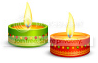Saral Gyan team celebrates this festive season offering discounts upto 30% & valuable freebies. Click here for details.SARAL GYAN DUSSEHRA - DIWALI OFFER 2019MULTIBAGGER SMALL / MID CAPS & LONG TERM INVESTING REPORT >>>  FREE DOWNLOAD

TECHNO-FUNDA (POTENTIAL MULTIBAGGER) STOCK 2019 REPORT >>>  FREE DOWNLOAD

PAST PERFORMANCE >>> HIDDEN GEMS, VALUE PICKS & WEALTH-BUILDER >>>  VIEW / DOWNLOAD

SUBSCRIPTION:        FREE SUBSCRIPTION      |      PAID SUBSCRIPTION     |      PAYMENT OPTIONS

How to Calculate & Evaluate CAGR?

CAGR stands for “Compound Annual Growth Rate”

It is the growth rate of a company expressed on an annualized basis. This also takes into consideration the effect of compounding. Let’s look at an example to understand it better.

Say the sales of a company 4 years back was 100. Today, after 4 years, it is 200. A simple conclusion is that sales has increases by 100% in 4 years. But does it mean that it has increased by 25% each year?

That would not be correct, as simply dividing 100% by 4 doesn’t take into consideration the compounding effect.

So, to find out the per year growth rate of a company, we use the compound interest formula.

A = P * ( ( 1 + r ) ^ n )

Where

A = Final Amount
P = Principal amount
r = Rate of interest, expressed in %
n = Number of years

In our example,

A = 200
P = 100
r = The annual growth rate (that we want to find out)
n = 4 years

From the above formula, we find out that r is around 19%.

How to interpret?

It means that the average growth of the company over these 4 years, taking into account the impact of compounding, is 19%.

In the first year, the company grew from 100 to 119. In the second year, it grew 119 to 142. In the third year, it grew by 19% from 142 to 168.5, and in the fourth year, it grew from 168.5 to 200.

Other Thoughts

This principle is very important to understand, because it is used at many places.

For example, it is looked at while examining at returns generated by mutual funds (MFs). Whenever one sees returns for more than 1 year, they are expressed in terms of CAGR. If they are not expressed in CAGR terms, the returns are not accurate!

CAGR should also be used while considering any investment. Just take the example of the Bhavishya Nirman Bonds issued by NABARD.

These have been issued at around Rs. 9750. The investment is for 10 years, after which the investor gets back Rs. 20000.

Thus, the return is Rs. 20000 – Rs. 9750 = Rs. 10250, or 105% in 10 years.

Does this mean that the return is 10.5% per year?

No! It has to be calculated using the CAGR formula, where:

A = 20000
P = 9250
r = Rate of return to be found out
n = 20 years,

Using the formula above, we find out that the rate is actually 7.5% per annum. Now, that’s quite far from 10.5%.